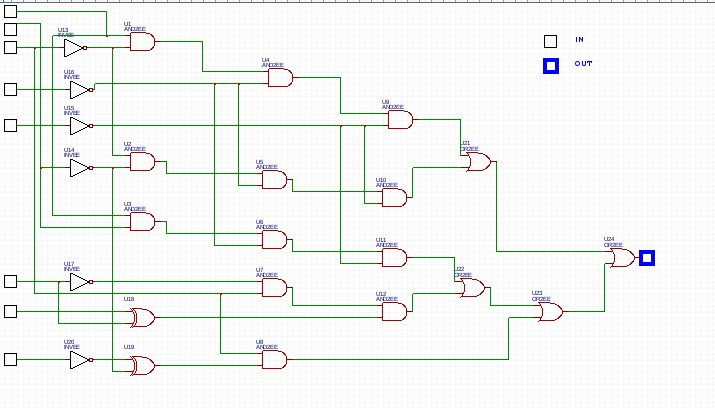# Nuit du hack quals 2016 - Invest

We received a invest.pcapng file which contains a schematic, encrypted files and a binary chain. Once the binary chain was passed in the schematic we obtained a password to decrypt with OpenSSL the files. The resulting file is a Word document with the Flag inside.

### Description

A paranoid guy seems to have secured his file very well. But I am convinced he made a mistake somewhere.

### Details

Points: 50

Category: Inforensic

Validation: 70

### Solution

The challenge provided us with invest.pcapng, a packet capture for us to analyze. With Wireshark we could extract several files with File->Export Objects->HTML. Within the pcap is a file called ‘key.txt’, which contains a binary chain. Interpreting the chain in ASCII showed that it does not look really random:

G^cnI9^GG9G9G9G9^cnInI95^c95nInIG^95nI^cG^95^c^c^cG^^cnIG^95G^nI^c^cnI^c^c95G^^c^c^cG^G^^cnInI^c

The pcap also contains several pictures; one in particular seems interesting:We noticed that this function takes 8 bit as input and output one bit. Among the downloaded they were 81 files with their name starting by encrypt. They are base64 encoded. We merged, decoded them and obtained a file which start with the string Salted__.

> binwalk merged.bin

--------------------------------------------------------------------------------
0             0x0             OpenSSL encryption, salted, salt: 0x7DD883F026435AB8


It means it is a file encrypted with OpenSSL. Then we coded the function represented by the previous picture:

import binascii

key="010001110101111001100011011011100100100100111001010111100100011101000111001110010100011100111001010001110011100101000111001110010101111001100011011011100100100101101110010010010011100100110101010111100110001100111001001101010110111001001001011011100100100101000111010111100011100100110101011011100100100101011110011000110100011101011110001110010011010101011110011000110101111001100011010111100110001101000111010111100101111001100011011011100100100101000111010111100011100100110101010001110101111001101110010010010101111001100011010111100110001101101110010010010101111001100011010111100110001100111001001101010100011101011110010111100110001101011110011000110101111001100011010001110101111001000111010111100101111001100011011011100100100101101110010010010101111001100011"

chunks, chunkSize = len(key), 8
l = [ key[i:i + chunkSize] for i in range(0, chunks, chunkSize) ]

# The logic part

s = ""

for b in l:

b0 = int(b)
b1 = int(b)
b2 = int(b)
b3 = int(b
b4 = int(b)
b5 = int(b)
b6 = int(b)
b7 = int(b)

c1 = b0 and (not b2)
c2 = (not b2) and (not b1)
c3 = b0 and b1
c4 = b5 ^ b6
c5 = (not b1) ^ (not b7)

d1 = c1 and (not b3)
d2 = c2 and (not b3)
d3 = c3 and (not b3)
d4 = b2 and (not b5)
d5 = c5 and b2

e1 = d1 and (not b4)
e2 = d2 and (not b4)
e3 = d3 and (not b4)
e4 = d4 and c4

f1 = e1 or e2
f2 = e3 or e4

g = f2 or d5

o = int(g or f1)
s += str(o)



We passed the string contains in key.txt to the script and we obtained the string “4Ukz95F2YqPi”. The string is 12-byte long. We did not know any cipher using such length for the key so we thought about a password. Since the guy is paranoid he would have used a strong block cipher. We started with AES-128:

openssl enc -aes128 -in merged.bin -out merged.out -d  -k 4Ukz95F2YqPi -p
salt=7DD883F026435AB8
key=215350CECF73E345AF6894267B335AA0
iv =7413F91D4B87534B953CC656476C3107


Then we tried AES-256:

openssl enc -aes256 -in merged.bin -out merged.out -d  -k 4Ukz95F2YqPi -p
salt=7DD883F026435AB8
key=215350CECF73E345AF6894267B335AA07413F91D4B87534B953CC656476C3107

grep "NDH" -r merged.out_FILES/